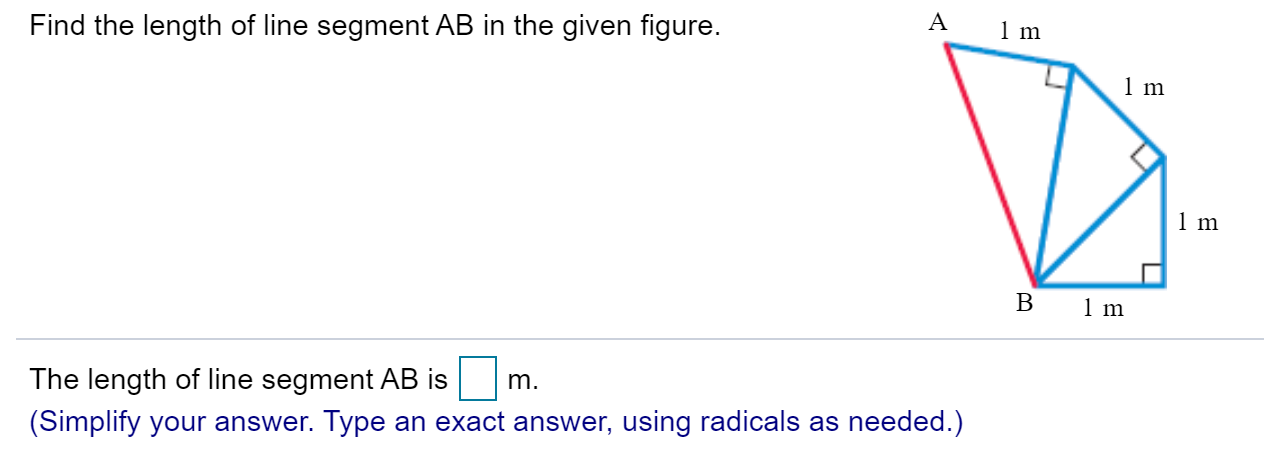# A1 mFind the length of line segment AB in the given figure.1 m1mВ1 mm.The length of line segment AB is(Simplify your answer. Type an exact answer, using radicals as needed.)

Question
8 viewshelp_outlineImage TranscriptioncloseA 1 m Find the length of line segment AB in the given figure. 1 m 1m В 1 m m. The length of line segment AB is (Simplify your answer. Type an exact answer, using radicals as needed.) fullscreen
check_circle

Step 1

First of all, label the all the triangles.

Step 2

Now, consider the ∆BCD.

Using Pythagoras theorem, find BD.

Step 3

Now, consider the ∆BDE.

Using Pythagoras th...

### Want to see the full answer?

See Solution

#### Want to see this answer and more?

Solutions are written by subject experts who are available 24/7. Questions are typically answered within 1 hour.*

See Solution
*Response times may vary by subject and question.
Tagged in

### Triangles